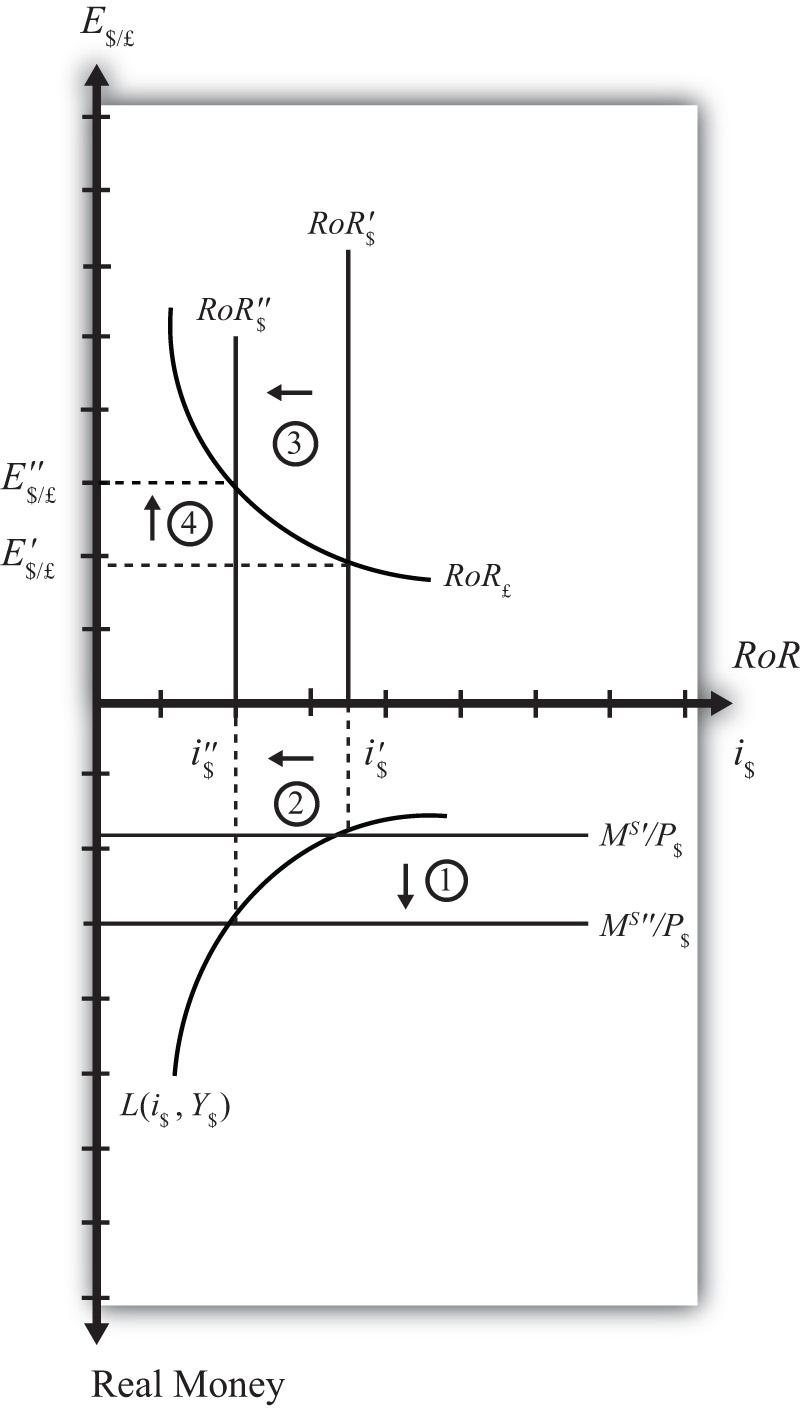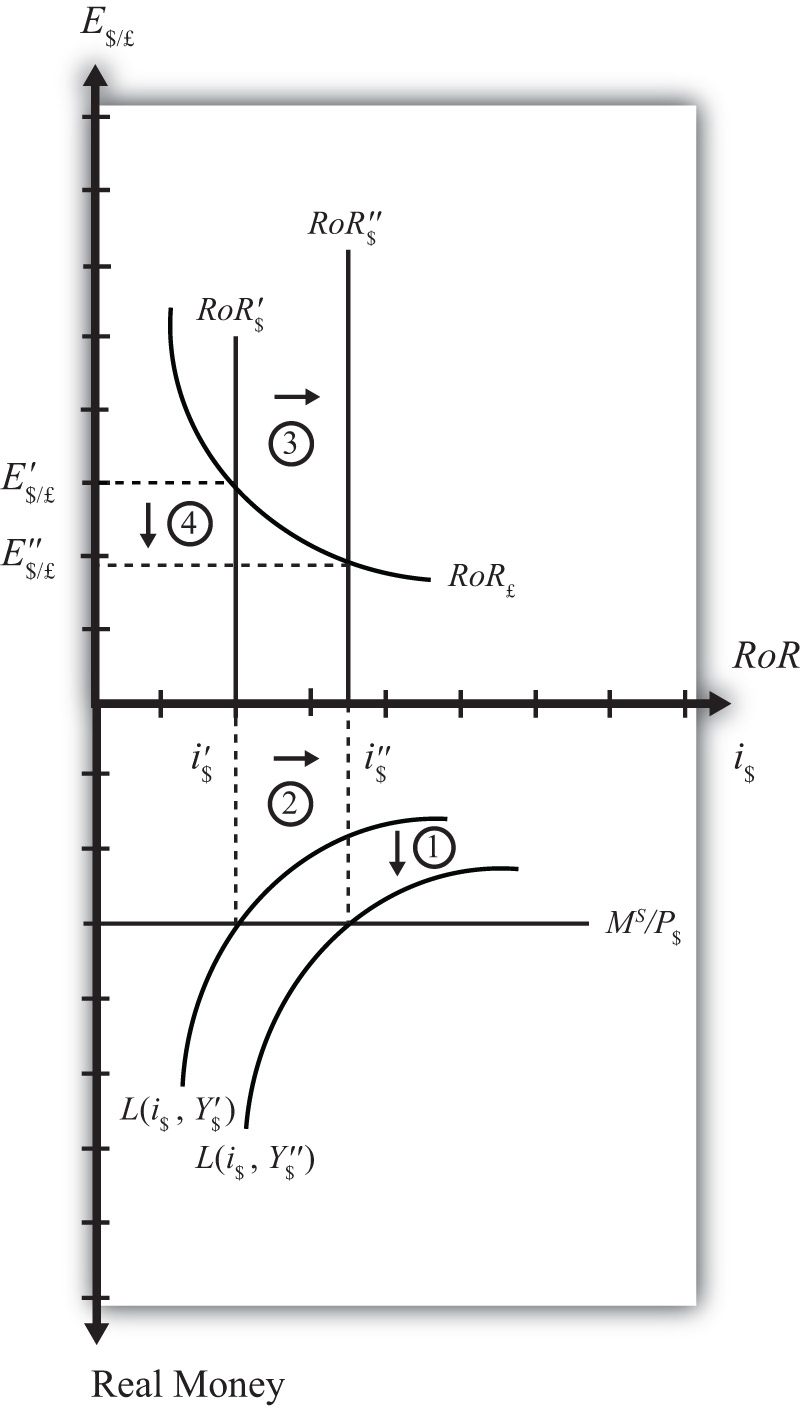## 7.13 Comparative Statics in the Combined Money-Forex Model

### Learning Objective

1. Show the effects of an increase in the money supply and an increase in GDP on the interest rate and exchange rate using the two-quadrant money-Forex market diagram.

Comparative statics is any exercise examining how the endogenous variables will be affected when one of the exogenous variables is presumed to change, while holding all other exogenous variables constant. Holding other variables constant at their original values is the “ceteris paribus” assumption. We will do several such exercises here using the combined money-Forex market diagram.

## An Increase in the U.S. Money Supply

Suppose the U.S. money supply increases, ceteris paribus. The increase in MS causes an increase in the real money supply (MS/P\$), which causes the real money supply line to shift “down” from MS′/P\$ to MS″/P\$ (step 1) in the adjacent Money-Forex diagram, Figure 7.9 "Effects of an Increase in the Money Supply". (Be careful here: down in the diagram means an increase in the real money supply.) This causes a decrease in the equilibrium interest rate from i\$′ to i\$″ (step 2). The decrease in the U.S. interest rate causes a decrease in the rate of return on dollar assets: RoR\$ shifts from RoR\$′ to RoR\$″ (step 3). Finally, the reduction in the dollar rate of return causes an increase in the exchange rate from E\$/£ to E\$/£ (step 4). This exchange rate change corresponds to an appreciation of the British pound and a depreciation of the U.S. dollar. In summary, an increase in the U.S. money supply, ceteris paribus, causes a decrease in U.S. interest rates and a depreciation of the dollar.

Figure 7.9 Effects of an Increase in the Money Supply## An Increase in U.S. GDP

Suppose there is an increase in U.S. GDP, ceteris paribus. This will increase real money demand, causing a “downward” shift in the real money demand curve from L(i\$, Y\$′) to L(i\$, Y\$″) (step 1) in the Money-Forex diagram, Figure 7.10 "Effects of an Increase in GDP". (Remember, real money increases as you move down on the rotated money diagram.) This causes an increase in the U.S. interest rate from i\$′ to i\$″ (step 2). The increase in the interest means that the rate of return on dollar assets increases from RoR\$′ to RoR\$″ (step 3). Finally, the increase in the U.S. RoR causes a decrease in the exchange rate from E\$/£ to E\$/£ (step 4). The exchange rate change corresponds to an appreciation of the U.S. dollar and a depreciation of the British pound. In summary, an increase in real U.S. GDP, ceteris paribus, causes an increase in U.S. interest rates and appreciation (depreciation) of the U.S. dollar (British pound).

Figure 7.10 Effects of an Increase in GDP### Key Takeaways

• In the money-Forex model, an increase in the U.S. money supply, ceteris paribus, causes a decrease in U.S. interest rates and a depreciation of the dollar.
• In the money-Forex model, an increase in real U.S. gross domestic product (GDP), ceteris paribus, causes an increase in U.S. interest rates and appreciation (depreciation) of the U.S. dollar (British pound).

### Exercise

1. Using the Forex market and money market models, indicate the effect of each change listed in the first row of the table, sequentially, on the variables listed in the first column. For example, “Expansionary U.S. Monetary Policy” will first cause an increase in the “Real U.S. Money Supply.” Therefore, a “+” is placed in the first box of the table. In the next row, answer how “U.S. Interest Rates” will be affected. You do not need to show your work. Note E\$/* represents the dollar/foreign exchange rate. Use the following notation:

+ the variable increases

the variable decreases

0 the variable does not change

A the variable change is ambiguous (i.e., it may rise, it may fall)

Expansionary U.S. Monetary Policy An Increase in U.S. Price Level An Increase in U.S. Real GDP
Real U.S. Money Supply +
U.S. Interest Rates
RoR on U.S. Assets
Foreign Interest Rates
RoR on Foreign Assets
U.S. Dollar Value
E\$/*